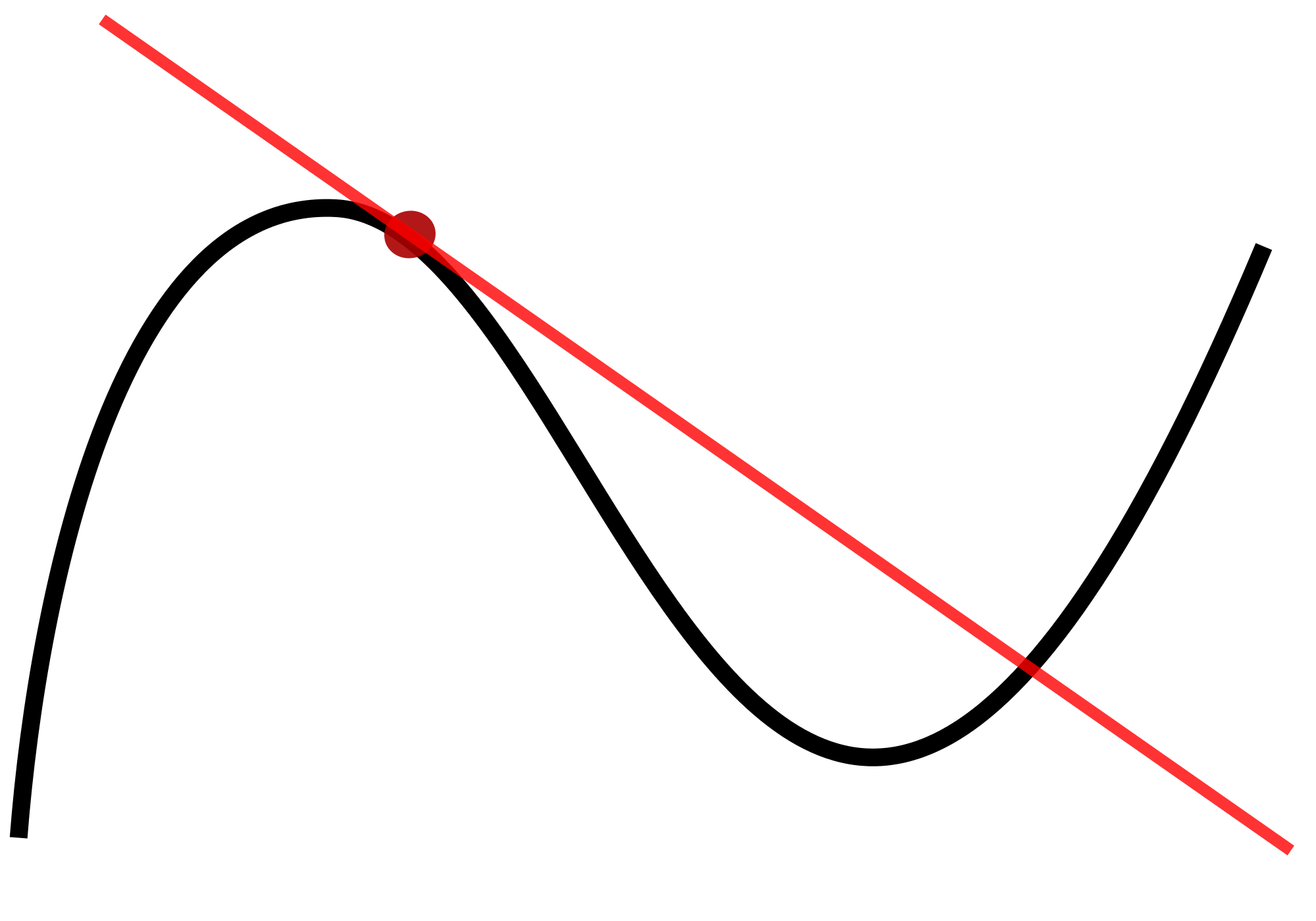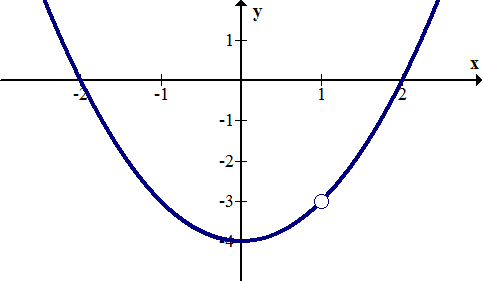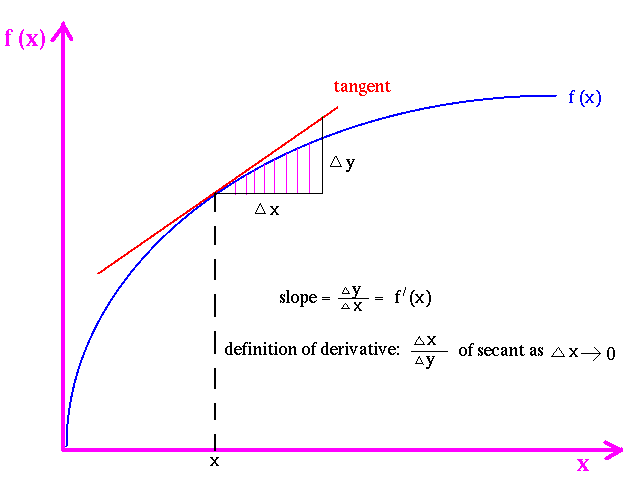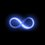# VE: Limit-Definition of DerivativeUnsatisfied with blocks of mathematical jumbo theorems and proofs of the limit-definition of derivative? Confused when you plug in numbers closer and closer to the desired value - yet never the value itself - and then your teacher claims the limit is EXACT? Maybe you just have a gut-feeling that it's right, but not a brain-feeling - because the concept was dumped on you as true and correct and you were forced to accept it. Either way, I shall provide a crystal-clear explanation of how and why the derivatives REALLY work - in this episode of Visual Explanations. Let's dive:

$\huge\lim_{\Delta x \rightarrow0}{\frac{f\left(x+\Delta x\right)-f(x)}{\left(x+\Delta x\right)-x}}$

Above lies the ULTIMATE EXPRESSION. We shall now tear it apart and pierce into the heart of Calculus.

1. Punching Holes

Remember the holes in functions? What are they and how can we make some for ourselves? If you don't know what holes are, click here. Now let's make some.

We can punch a hole at any desired point $A$ in a function by multiplying the function by $\frac{x-A}{x-A}$. This way, at $x=A$, we get $0/0$ - and thus a hole. In a graphing calculator that plots holes, you can draw a bracelet by punching a bunch of holes into $x^2$ ;)

For example, imagine the graph $x^2-4$. Let's punch a hole at $x=1$. How do we do it? Hole in $f(x)$ at $A$ do $\left(\frac{x-A}{x-A} \right) f(x)$. So,

$f(x)=(x^2-4)\left(\frac{x-1}{x-1}\right)$BOOM! We can keep adding holes - at $x=$whatever - I just don't have a graphing calculator that plots holes to demonstrate. Hope you get the idea.

2. Filling Holes

So why do you begin Calculus with the POINTLESS practice of solving $0/0$ limits? Besides sharpening your Algebra, it is to help you conquer the DERIVATIVE. And then, you learn a bunch of differentiation rules, and completely forget about the limit-definition of derivative. Well done, public school systems. So let's fill some holes.

~~~~~Solve:

$\displaystyle \lim_{x\rightarrow 2}{\frac{4x^4-11x^3+8x^2-5x+2}{x-2}}$

Gotcha here:

$\displaystyle \lim_{x\rightarrow 2}{\frac{(4x^3-3x^2+2x-1)(x-2)}{x-2}}$

And thus the answer is $4(2)^3-3(2)^2+2(2)-1=23$. So who cares? What did we just find? Your teacher will tell you "this is the point the function approaches, but never touches, around $x=2$." Cool. So? "So, let's take out our calculators and check if this is right. Let's start at $x=1.9$... ... Now we'll come from the right - start with $x=2.01$..." ...

In your mind you're thinking "Well alright, we seem to be approaching this particular point. But how do we know if that is EXACTLY what we are approaching? For example, what if it's 22.99999....314159 instead?"

Well it's not. It is EXACTLY 23. How do we know?

We know because someone secretly punched a hole through that function. Yeah - that $\left(\frac{x-2}{x-2}\right)$. He's a nasty one. He's a sneaky one. He makes it appear as if everything is alright - all the time - until you get to $x=2$. Then, you find out that somebody robbed the function! The point is GONE!! So what do you do? You fill the hole. How? By assuming that $\left(\frac{x-2}{x-2}\right)$ was never there to begin with. THIS IS THE GOLDEN KEY. All we're doing by getting rid of $0/0$ in any function is obtaining the function prior to it being modified on that specific point. Notice if you remove the hole, the rest of the function is 100% the same. Essentially, all we're doing is undoing our little "Punching Holes" operation. Take $f(x)$. Punch a hole through it. Now no matter what, you cannot evaluate the function at that point you punched through. So what do you do? You get rid of the thing that makes the function at that point - the $0/0$ - and evaluate it so. Now you know EXACTLY what that function is "approaching" - it is approaching whatever it would be approaching if the hole was not there in the first place. And finding THAT is as easy as following criminal footsteps right to... the criminal ;)

3. Limit-Definition of Derivative

Look up at the majestic expression. Let me put it here again:

$\large\lim_{\Delta x \rightarrow0}{\frac{f\left(x+\Delta x\right)-f(x)}{\left(x+\Delta x\right)-x}}$

So notice the first thing - I did not write the expression it its reduced form - that is, with just $\Delta x$ on the bottom. $\large{\text{I ABSOLUTELY HATE WHEN TEACHERS/TEXTBOOKS DO THAT!!!}}$ From now on, NEVER write this expression that way! Yuck.

Why the big fuss? Cause - by simplifying the formula, you completely and absolutely obliterate the quintessential key component of the entire expression - the $(x+\Delta x)-x$. We thus forget what it actually stands for - which is, the limit expression for the SLOPE OF THE SECANT LINE OF THE FUNCTION! All this is is $\frac{y_2-y_1}{x_2-x_1}$ written in a fancy form - that's it! But a mere $\Delta x$ on the denominator just completely scratches $x_2-x_1$ out of the picture. Unacceptable. See image below:Now, let us answer the following question:

Why does the limit-definition of derivative expression yield the EXACT instantaneous rate of change / derivative of the function?

First, realize again what the expression stands for: the slope of the secant line of the function between points $x$ and $x+\Delta x$. Further realize that the slope of the secant line between any two points is the AVERAGE RATE OF CHANGE of the function between those two points. ${}^{1}$ By letting $\Delta x$ go to zero, we're aiming for the INSTANTANEOUS RATE OF CHANGE at a SINGLE POINT. At first this seems to even make little sense, but graphically you can see how when $\Delta x$ approaches zero, and the two points become one, the secant line is now a tangent line and represents the rate of change of the function at the desired point:$\small{\text{(1-Think of it this way: speed=distance/time. Replace speed with rate of change, distance with}}$ $\small{\text{change, and time with interval over which change occurs. So, if we cover 4 meters in 2 seconds,}}$ $\small{\text{we're traveling at 2 meters / second. Or, if our function changes 4 units from point}}$ $\small{a}$ $\small{\text{to point}}$ $\small{a+2,}$$\small{\text{then the average rate of change between those two points will be 2.)}}$

To REALLY${}^{2}$ understand this, we need to understand the Antiderivative. But that's for the next VE. For now, you get the gist. Back on task. So, why does the formula work? Well, now that we know what the formula stands for, we may put it in its ugly form:

$\large{\lim_{\Delta x \rightarrow0}{\frac{f\left(x+\Delta x\right)-f(x)}{\Delta x}}}$

If you've ever done derivatives, you know we're looking to factor out $\Delta x$ on the top and cancel it with the bottom. Ah-ha - we're filling the hole, just like in all other limits, by defining the point of expression with the removal of $0/0$! That's it.

Let's re-cap: The expression for the Limit-Definition of Derivative is the one for a secant line, which represents the average rate of change between any $x$ and $x+\Delta x$. When we allow $\Delta x$ to go to $0$, we are searching for the instantaneous rate of change of the function at some point $x$ (by just leaving it $x$, we derive the general expression - the derivative function $f'(x)$ for any $x$ we desire. It is the derivative function.) Initially, we cannot do this because the expression for the slope (the rate of change) is . We resolve this issue by realizing that if we remove $\frac{\Delta x}{\Delta x}$ from the expression, we allow ourselves to evaluate the expression at that point and get the exact result - as if there were never a hole to begin with in the first place.

That's it.Note by John M.
5 years, 10 months ago

This discussion board is a place to discuss our Daily Challenges and the math and science related to those challenges. Explanations are more than just a solution — they should explain the steps and thinking strategies that you used to obtain the solution. Comments should further the discussion of math and science.

When posting on Brilliant:

• Use the emojis to react to an explanation, whether you're congratulating a job well done , or just really confused .
• Ask specific questions about the challenge or the steps in somebody's explanation. Well-posed questions can add a lot to the discussion, but posting "I don't understand!" doesn't help anyone.
• Try to contribute something new to the discussion, whether it is an extension, generalization or other idea related to the challenge.
• Stay on topic — we're all here to learn more about math and science, not to hear about your favorite get-rich-quick scheme or current world events.

MarkdownAppears as
*italics* or _italics_ italics
**bold** or __bold__ bold
- bulleted- list
• bulleted
• list
1. numbered2. list
1. numbered
2. list
Note: you must add a full line of space before and after lists for them to show up correctly
paragraph 1paragraph 2

paragraph 1

paragraph 2

[example link](https://brilliant.org)example link
> This is a quote
This is a quote
    # I indented these lines
# 4 spaces, and now they show
# up as a code block.

print "hello world"
# I indented these lines
# 4 spaces, and now they show
# up as a code block.

print "hello world"
MathAppears as
Remember to wrap math in $$ ... $$ or $ ... $ to ensure proper formatting.
2 \times 3 $2 \times 3$
2^{34} $2^{34}$
a_{i-1} $a_{i-1}$
\frac{2}{3} $\frac{2}{3}$
\sqrt{2} $\sqrt{2}$
\sum_{i=1}^3 $\sum_{i=1}^3$
\sin \theta $\sin \theta$
\boxed{123} $\boxed{123}$

## Comments

Sort by:

Top Newest

This is a nice note.

I personally agree that the derivative definition looks more comprehensible when you put it in the ((x+dx)-x) way.

- 5 years, 10 months ago

Log in to reply

I have owed this to you for a while now, @Agnishom Chattopadhyay . Sorry for the delay. Hope you find it somewhat useful ;) Some feedback would be appreciated!

Up next is my greatest breakthrough in understanding Calculus - the Antiderivative - the Integral. That one is going to take a while, but I'll make it real good. Until then, thanks for reading my stuff! @Followers ^.^

Oh and btw - IF ANYTHING IS UNCLEAR, YOU BETTER ASK ME - OR I'LL HAUNT YOU ON EXAMS!!! @Followers

- 5 years, 10 months ago

Log in to reply

@John Muradeli Please post the antiderivative note.

This note is too good.

- 4 years, 11 months ago

Log in to reply

I will say this for right now. Given any function $f(x)$, the following is true

$f(x+\Delta x)-f(x)=\frac { 1 }{ 1! } f'(x)\Delta x+\frac { 1 }{ 2! } f''(x){ \Delta x }^{ 2 }+\frac { 1 }{ 3! } f'''(x){ \Delta x }^{ 3 }+...$

so that the right side is always divisible by $\Delta x$, and so there's never a problem with any division by $0$. But I agree with you that it's a philosophically unsatisfying approach, and it's a circular argument to begin with. Your approach is at least more interesting, and probably better.

What makes these kinds of arguments relevant is in field of Finite Element Analysis, which is the driving force behind computer simulations of physics and engineering. Details such as this does matter significantly in FEA work, and departs from methods of "Real Analysis" in calculus. That is, many aspects of "Real Analysis" in calculus seem to have little or no bearing on FEA work in the industry.

- 5 years, 10 months ago

Log in to reply

Thanks.

The true reason why I came up with this note is because absolutely none of the book/instructor explanations rung any clearview bell for me as to why the limits are EXACT and how the limit-definition of derivative produces the instantaneous rate of change of a function. You see, the thing you typed up there - if I saw that the first time I was learning calculus, it'd look to me like those equations I put down for coming up with that fake model for fruitfly behavior - I think you remember. It just looks like JUMBO. My goal was to come up with something simple, yet satisfactory at explaining the fundamental idea. And I think I've done pretty well in that respect. To me, personally, all those "approximation" methods are very misleading - for they paint a picture in a learner's mind that the limit is never exact - but simply "infinitely close." And I've had this view for a looong time.

But my real headbanger didn't come up until I've learned the integral. It has ALWAYS bugged me as to why when we integrate x^2 we have to divide by 3 to obtain area under the curve. It made sense when we integrate x to get x^2/2, because that's just half a rectangle - which graphically makes sense. But then I realized that this thinking is useless - for we can not at all use it to explain why the area under the curve of $\sin{(x)}$ is $-\cos{(x)}$. Then I realized it's time to think antiderivatives - for all areas of all forms. And after a few months of occasional intense scrutiny, I've done it. And I will soon make another VE dedicated to this matter.

- 5 years, 10 months ago

Log in to reply

Oh and VE stands for Visual Explanation - as implied in the intro. I guess, though, in some subtle way, you could argue this to be Victory in Europe, for European mathematics > USA mathematics ;)

- 5 years, 10 months ago

Log in to reply

For The Historically Challenged- 5 years, 10 months ago

Log in to reply

OLD.

- 5 years, 10 months ago

Log in to reply

And I can still beat you going up vertical rock. Want to try?

- 5 years, 10 months ago

Log in to reply

Hi, John. Nice article :)

I may sound like I am rioting. But, I am not.

I have just tried to comment on the "EXACT" nature of limits (and why it's not exactly exact). Hope you will find it helpful =)

Some symbolic representation are better because they reflect the underlying procedure more clearly. For e.g., as you have pointed out, the limit representation of first order derivative is better with $x+ \Delta x$ in the denominator than only $\Delta x$.

Similarly some procedures are better in the sense that they reflect the use of underlying concepts in a better way.

The representation $\displaystyle \lim_{x\to 2} \frac{ x^{2}- 2^{2} }{x-2}=4$ leads to misunderstanding because of the symbol "$=$". It says that limit of the given function when $x$ tends to $2$ "is" $4$. As if the limit is "Exactly" $4$. But, strictly speaking, it is not exactly $4$.

In place of "$=$", we must substitute "$\to$" so that we get something like this: $\displaystyle\lim_{x\to 2} \frac{ x^{2}- 2^{2} }{x-2} \to 4$. The problem with "$=$"is that it obscures the fact that the limit tends to $4$ or approaches $4$ but is never exactly $4$. The "but..." is indispensable.

That was with symbols. Now, lets look at the procedure: $\displaystyle \lim_{x\to 2} \frac{ x^{2}- 2^{2} }{x-2} = \lim_{x\to 2} \frac{(x-2)(x+2) }{x-2}= \lim_{x\to2}x+2 =4$. Now, the question is where did we use the fact that the function $\displaystyle \frac{ x^{2}- 2^{2} }{x-2}$ is indeterminate at $x=2$ and so we cannot simply plug the value $x=2$ in the given function?

Consider another approach: Since we cannot substitute $x=2$, lets substitute $x=2+h$, where $h$ is any number other than $2$. Now, it totally depends on $h$ how much $2+h$ will differ from $2$, ($2+h - 2$=?). Notice that the only number to make the difference negligible isn't a negative number nor a positive number but zero! (Actually there is no difference if $h=0$, but the use of the word "negligible" seemed more appropriate to our discussion). And yet we can't have $h=0$. So what number should we assign to $h$ to make the difference negligible?

(At this point, someone may worry about why we are making the difference negligible. Really why? Because that is the very purpose of limit! What is the purpose of limit? To find limiting values. Why do we need to find limiting values? Because while investigating a function we may come across a point where it is not possible to progress further through plug & play...Lets not extend further to : Why are we investigating a function? Why are we studying Mathematics? More importantly, what is a function doing in Mathematics? =D)

So, to overcome the difficulty of being unable to directly assign $h=0$ , we allow $h$ to be any number and then impose that it can approaches zero. That is, it can attain any value as much close as possible to zero, but not exactly zero ($h=000.1$ or $h=00000000.1$ but $h \ne 0$ ). Symbolically, the same things can be represented (& summed up) as: Let $x=2+h$ where $h \to 0$ but $h \ne 0$.

Then, we can write,

$\displaystyle \lim_{h\to 0} \frac{ (h+2)^{2}- 2^{2} }{(h+2)-2} \to 4$. Now, this approach does take into account that we haven't directly substituted $x=2$, anywhere in the function, to find the limit.

We can't say that the limiting value is 'exactly' $4$.

- 5 years, 10 months ago

Log in to reply

Wassup M.Philic!!

So, aright I see your reasoning. I will, however, have to disagree. I too at first scrutinized the equal sign. However, it all stems from mathematical definition. BY DEFINITION, when applying the "lim" operation to a function, it is automatically transcribed into "approaching." The equal sign, logically, stands for "is" - rather than equals. So, in other words, it is saying "the limit of the function as the input parameter approaches the chosen value IS ..." - now, grammatically, all you need to do is trim the fat - and the fat is all that follows "limit." So, trimming, we get "The limit IS..." See? And when you define "limit," it makes total sense.

Now to your point of it not being EXACT. From your reasoning I can reasonably assume that you are having the exact same issue I have had prior to my VE discovery. But then it appears my VE resolved nothing! But let's not haste: what is it that you think is not "exact," here? I have demonstrated that by canceling $0/0$ you basically obtain the exact same function except now it is defined at the desired point. This makes it possible for us to PLUG IN DIRECTLY INTO THE FUNCTION, and OBTAIN THE EXACT VALUE.

Did I miss anything?

- 5 years, 10 months ago

Log in to reply

What I meant to say is that when we say $x$ is approaching $2$, it means there is no unique value of $x$. That, $x$ can be $2.00001$ or $x=1.999999999999$ or $x$ can be $-27$ ! Since we are interested in approaching value, we don't consider cases, like $x=-27$, that take us far away from our main purpose( i.e. to investigate a function "at" $x=a$, where the function is indeterminate when $x=a$ ) Similar things happens to "limit" of a function.

- 5 years, 10 months ago

Log in to reply

Oh yes of course. But like I've argued, I hate those demonstrations for they are very misleading and can make people think that the limit is only a very accurate - but not an EXACT - value towards which a function approaches given a particular input value.

- 5 years, 10 months ago

Log in to reply

Oh! Yes, now I get it. Since you were explaining 1st order derivative in terms of limit, it' misleading to view limits as "not exact".

If I try to teach students that tangent of a straight line is never one but approaches one, then surely there will be a mutiny. I may even have to walk the plank

:D

Thanks for the clarification

- 5 years, 10 months ago

Log in to reply

Right right - and I personally myself could not find an explanation ANYWHERE that clearly demonstrated how the derivative is EXACT, not just VERY VERY ACCURATE. For me, only my method works. And I hope it adds a way of thinking to your repertoire as well ^.^

- 5 years, 10 months ago

Log in to reply

You think lines to connect directions a to b in stead of a to b think all directions as forward motion following a spherical dimensional path to origin a origin of a traveling from position 1 = 1 Or 0-1o8O8O8o1 -0 IN OTHER WORDS 2INFINITE TO ot finite to TO INFINITE 224.12 1 1 =2 2 2=4 4 4=8 1x 1y one male one female one proton one neutron 1 PRO + 1 NEU= 2 Electrons pro 1 electron neu 1 electron the pair one of two P+N pair two of two P+e pair three of two N+e 22 You know a uneak life form is the worm it from its self can produce it's self so theoreticly atoms of them self's can produce of them self's allowing for mass infinitely creating from within there own nucleus with each other really pro are inner changeable so to speak as well as neu which allows for infinite compasiy as it yet and thus the reason for this activity is simply place of origin of diversity. Truly compelling and admersively intelligently designed and crafted by one .

- 5 years, 7 months ago

Log in to reply

×

Problem Loading...

Note Loading...

Set Loading...